# Scanner - Elasticity of Demand (2009-2018) (Theory of Consumer Behaviour) Notes | Study Economics Class 11 - Commerce

## Commerce: Scanner - Elasticity of Demand (2009-2018) (Theory of Consumer Behaviour) Notes | Study Economics Class 11 - Commerce

The document Scanner - Elasticity of Demand (2009-2018) (Theory of Consumer Behaviour) Notes | Study Economics Class 11 - Commerce is a part of the Commerce Course Economics Class 11.
All you need of Commerce at this link: Commerce

N.C.E.R.T Questions

(Q1) Define price elasticity of demand

(Q2) What are three distinct cases where elasticity of demand is the same at all points of the demand curve ?

(Q3) Draw diagram showing elasticity equal to (a) zero (b) one and (c) infinity .

(Q4) Draw a straight line demand curve. Choose any three points on it and compare the point elasticities at these three points

(Q5) The price elasticity is .5. The % change in quantity is 4. What is the % change in price ?

(Q6) “ If a product price increases , a family’sspending on the product has to increase “ . Defend or Refute

or
Show effect of an increase in price on total expenditure depending on the value of price elasticity

(Q7) A dentist was charging Rs 300 for a standard cleaning job and per month it used to generate total revenue equal to Rs. 30000. She has since last month increased the price of dental cleaning to Rs 350. As a result fewer customer are now coming for dental cleaning, but total revenue is now Rs. 33250. From this what can be concluded about the Ed for such a dental services ?

(Q8) Suppose the price elasticity of demand is - . 2. How will the expenditure on the good be affected if there is a 10 % increase in the price of good ? (Ans increase)

(Q9) What is the relationship between slope and elasticity of demand ?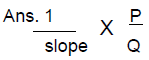(Q10) Demand for the product is elastic. Its price falls. What will be its effect on total expenditure on the product ? Give numerical example ?

C.B.S.E Questions

(Q1) Mention any three points that affects price elasticity of demand of a commodity ?

(Q2) Explain the total expenditure method of measuring price elasticity of demand of a commodity ? When demand is said to be inelastic ?

(Q3) Explain with the help of diagram the geometric method of measuring price elasticity of demand

(Q4) The market demand for good at Rs 4 is 100 units,. The price rises and as a result its market demand falls to 75 units. Find out the new price if Ed is (-) 1

Ans: Rs 5

(Q5) A 5% fall in price of X leads to 10% rise in demand for X. A 2% rise in price of Y leads to 6% fall in demand for Y. Calculate Ed of demand for X and Y ?

Ans: 2 , 3

(Q6) When the price is Rs 20, its Q.D is 800 units. When its price rises by Rs 5, Q.D falls by 20 %. Calculate Ed. Is demand elastic ?

Ans: 0.8

(Q7) A consumer spends Rs.250 on a good when its price is Rs 5 per unit. When the price rises to Rs 6 per unit, he spends Rs 240. Calculate Ed by percentage method

Sample Paper + C.B.S.E 2008 Question’s

(Q1) A consumer buys 100 units of a good at a price of Rs. 5 per unit. When price changes he buys 140 units. What is the new price if price elasticity of demand is - 2 ? (4)

Ans: rs 4

(Q2) A rise in the price of a good results in an increase in expenditure on it. Is its demand elastic or inelastic ?

(Q3) When price of a good falls from Rs. 5 to Rs. 3 per unit, its demand rises by 40 percent.Calculate its price elasticity of demand

Ans: ed = 1

(Q4) Price elasticity of demand of a good is (-)1. At a given price the consumer buys 60 units of the good. How many units will the consumer buy of the price falls by 10 percent ?

Ans:  66 units

(Q5) Price elasticity of demand of a good is (-)3. If the price rises from Rs. 10 per unit to Rs. 12 per unit, what is the percentage change in demand?

Ans: % fall in demand 60 % C

C.B.S.E 2009

(Q1) Explain the geometric method of measuring price elasticity of demand.(3)

(Q2) Explain any two factors that affect the price elasticity of demand of a commodity.(3)

CBSE 2010 + Sample Paper Questions

(Q1) When is the demand for a good said to be perfectly inelastic ?(1M)

(Q2) Explain the effect of the following on the price elasticity of demand of a commodity ?(3M)

(i) Number of substitutes
(ii) Nature of the commodity

(Q3) When price of a commodity falls by Re. 1 unit, its quantity demanded rises by 3 units. Its price elasticity of demand is (-) 2. Calculate its quantity demanded if the price before the change was Rs. 10 per unit.      (4M)

Ans:  15 units

(Q4) The price elasticity of demand of a commodity is (-) 1.5. When its price falls by Re. 1 per unit its quantity demanded rises by 3 units. If the quntity demanded before the price change was 30 units, what was the price at this demand ? Calculate.           (4M)

Ans: rs 14

(Q5) Quantity demanded of a commodity rises by 6 units when its price falls by Re. 1 per unit. Its price elasticity of demand is (-)1. If the price before the change was Rs. 20 per unit, calculate quantity demande at this price.        (4M)

Ans:  120 units

(Q6) What happens to total expenditure on a commodity when its price falls and its demand is price elastic ?(1 M)

(Q7) How does the nature of a commodity influence its price elasticity of demand?(3 M)

(Q8) The diagram shows AE is the demand curve of a commodity. On the basis of this diagram, state whether the following statements are true or false. Give reasons for your answer :                             (4 M)

(a) Demand at point B is price inelastic.

(b) Demand at point C is more price elastic than at point B.

(c) Demand at point C is price elastic.
(d) Price elasticity of demand at point C is greater than the
price elasticity of demand at point “D”

(Q9) Calculate the price elasticity of demand for a commodity when its price increases by 25% and quantity demanded falls from 150 units to 120 units.   (4 M)

Ans: ed = 0.8

(Q10) Calculate the percentage fall in demand for a good whose price rises from Rs. 10 per unit to Rs. 11 per unit. Its price elasticity of demand is -0.25. (4M)

Ans: 2.5 %

CBSE 2011 & CBSE 2012

(Q1) Why is demand for water inelastic .CBSE (D) 2010

(Q2) When price of a good is Rs.13 per unit, the consumer buys 11 units of that good. When price rises to Rs.15 per unit, the consumer continues to buy 11 units. Calculate Price Ed ?

Ans: ed = 0

(Q3) When price of a good is Rs.12 per unit, the consumer buys 24 units of that good. When price rises to Rs.14 per unit, the consumer continues to buy 20 units. Calculate Price Ed ?

Ans: ed = 1

(Q4) From the following data calculate price elasticity of demand :         (3 marks)
Price (Rs.)        Demand (units)
9                100
9               150

(Q5) A consumer buys 10 units of a commodity at a price of Rs. 10 per unit. He incurs an expenditure of Rs. 200 on buying 20 units. Calculate price elasticity of demand by the percentage method. Comment upon the shape of demand curve based on this information.           (4 marks)

Ans:  ed = infinite

(Q6) A consumer buys 8 units of a good at a price of Rs.7 per unit. When price rises to Rs. 8 per unit he buys 7 units. Calculate price elasticity of demand through the expenditure approach.Comment upon the shape of demand curve based on this information.

Ans:  ed = 1
(Q7) A consumer buys 14 units of a good at a price of Rs. 8 per unit. At price Rs.7 per unit he spends Rs.98 of the good. Calculate price elasticity of demand by the percentage method.Comment upon the shape of demand curve based on this information. (4 marks)

Ans:  ed = 0

Sample Paper 2014-15 & 15-16 & 16-17

(Q1) Comment upon the degree of elasticity of demand for Good X, in the following given situations, if the price of the commodity rises from Rs.5 per unit to Rs.7 per unit and the quantity demanded falls from 20 units to 16 units : (a) Using the total household expenditure method, (b) Using proportionate method.        (4M)

Ans: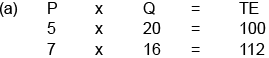Since the price & total expenditure carry positive relation Ed < 1, relatively inelastic demand.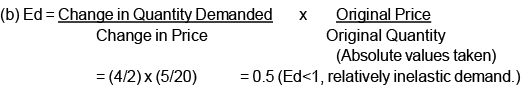(Q2) (a)  Arrange the following coefficients of price elasticity of demand in ascending order :

-0.7, -  0.3 , -1.1, - 0.8

(b) Comment upon the degree of elasticity of demand for Good X, using the total outlay method, if the price of X falls from 18 per unit to 13 per unit and its quantity demanded rises from 50 units to 100 units.

Ans: (a)  Ascending order: -0.3, -0.7,-0.8,-1.1.(minus sign only represents the inverse relation between price and quantity demanded)

(b) Ed > 1.

C.B.S.E & Sample Paper 2013

(Q1) When is the demand for a good said to be perfectly inelastic ?   (1 M)

Ans : When demand remains constant at all prices

(Q2) A perfectly elastic price-demand curve is parallel to the X-axis. Why or why not ?    (1 mark)

Ans: A perfectly elastic demand curve is parallel to the X-axis because the buyers are willing to buy any quantity of the  good at a given price.

(Q3) Price elasticity of demand for flowers and toys are respectively (-) 0.9 and (-) 0.5. Demand for which one is more elastic and why ?(3M)

(Q4) Explain the influence of following on price elasticity of demand of a good :(i) Substitute goods.(ii) Own price of the good.  (4 marks)

(Q5) A 5 percent fall in the price of a good raises its demand from 300 units to 318 units.Calculate its price elasticity of demand.    (4 M)

(Q6) When the price of a commodity falls by 20 percent, its demand rises from 400 units to 500 units. Calculate its price elasticity of demand. (4 M)

(Q7) Price elasticity of demand of a good is - 0.75. Calculate the percentage fall in its price that will result in 15 percent rise in its demand.(4 M)

C.B.S.E Paper 2014

(Q1) Give the meaning of ‘inelastic demand’.(1 M)

(Q2) When the price of a good falls from Rs.10 to Rs. 8 per unit, its demand rises from 20 units to 24 units. What can you say about price elasticity of demand of the good through the ‘expenditure approach’ ?       (3M)

Ans: As with fall in price, total expenditure also falls, price elasticity of demand is less than 1.Demand is inelastic. (No mark if the percentage method is used)

(Q3) When the price of a good rises from Rs.10 to Rs.20 per unit, its demand falls from 25 units.What can you say about price elasticity of demand of the good through the ‘expenditure approach’ ?  (3 M)

(Q4) A consumer buys 27 units of a good at a price of Rs.10 per unit. When the price falls to Rs.9 per unit, the demand rises to 30 units. What can you say about price elasticity of demand of the good through the ‘expenditure approach’ ? (3 marks)

(Q5) A consumer buys 18 units of a good at a price of rs 9 per unit  . The price elasticity of demand for the good is (-) 1 . How many units the consumer will buy at  a price of Rs 10 per unit

(Q5) A 20% fall in the price of a good raises its demand from 600 units to 750 units. Calculate its price elasticity of demand.

Ans: 16 units

(Q6) Price  ed = (-1) . . When its price per unit falls by one rupee , its demand rises from 16 to 18 units . Calculate the price before change ?

Ans: Rs 8

(Q7) Price elasticity of demand of two goods A and B is (-) 3 and (-) 4 respectively . Which of the two goods has higher elasticity and why ?

Ans: Good B has higher elasticity as compared to A. It is because with change in price by one percent, percentage change in demand for B is 4% while in case of good A it is only 3 percent.

C.B.S.E Paper 2015

(Q1) A consumer spends Rs. 100 on a good priced at Rs. 4 per unit. When its price falls by 25 percent, the consumer spends Rs. 75 on the good. Calculate the price elasticity of demand by the Percentage method.  (4 M)

Ans: ed = 0

(Q2) A consumer spends Rs.1,000 on a good priced at Rs. 10 per unit. When its price falls by 20 percent, the consumer spends Rs. 800 on the good. Calculate the price elasticity of demand  by the Percentage method.  (4 M)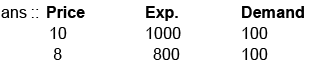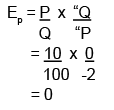(No marks if only the final answer is given)

(Q3) A consumer spends Rs. 400 on a good priced at Rs. 8 per unit. When its price rises by 25 percent, the consumer spends Rs. 500 on the good. Calculate the price elasticity of demand by the Percentage method. (4 M)

Ans ed = 0

(Q4) Comment upon the degree of elasticity of demand for Good X, in the following given situations, if the price of the commodity rises from Rs. 5 per unit to Rs.7 per unit and the quantity demanded falls from 20 units to 16 units :(i) Using the total household expenditure method,(ii) Using proportionate method.[SP (4M)]

(Q5) A consumer spends Rs.1,000 on a good priced at Rs.8 per unit. When price rises by 25 per cent, the consumer continues to spend Rs.1,000 on the good. Calculate price elasticity of demand by percentage method.(D)

(Q6) A consumer spends Rs. 60 on a good priced at Rs.5 per unit. When price falls by 20 per cent, the consumer continues to spend Rs. 60 on the good. Calculate price elasticity of demand by percentage method. (D)

Ans = - 1.25

CBSE 2016

(Q1) What will be the effect of 10 percent rise in price of a good on its demand if price elasticity of demand is (a) Zero (b) - 1 (c) - 2. (3M)

Ans: (a) Zero or no change (b) 10% fall (c) 20% fall              (1×3)

(Q2) Price elasticity of demand of good X is -2 and of good Y is -3. Which of the two goods is more price elastic and why ?   (3)

Ans: Y is more price elastic because one percent fall (rise) in price will lead to higher percent i.e. rise (fall) in demand. (or any other relevant explanation)

(Q3) Price elasticity of demand for the two goods X and Y are zero and (–) 1 respectively. Which of the two is more elastic and why ?

Ans: Y is more elastic than X because one percent change in price of good Y leads to one percent change in quantity demanded while one percent change in good X has no effect on demand of good X.

Drill Yourself

(Q1) At price Rs 10 , Q,D was 1000 units. The price changes to Rs 14 and Q.D 500 units.Calculate Ed

(Q2) At price Rs 50 , Q,D was 500 units. The price changes to Rs 60 and Q.D falls by 90 units.Calculate Ed and is its demand inelastic

(Q3) If the price elasticity for wheat is unit elastic and the consumer demands 40 kgs of wheat at a price of Rs 1 per kg. On what price the consumer will demand 36 kgs of wheat.

(Q4) When price of a commodity gets doubled , its quantity damanded gets reduced to half.Calculate the coefficient of price elasticity of demand ?

Ans : 0.5

(Q5) With rise in price from Rs. 8 to Rs.14, total expenditure on the commodity rises by 40% and becomes Rs.1,120. Calculate price elasticity of demand. Also indicate whether demand is elastic or inelastic.

(Ans. 0.267)

(Q6) The price elasticity of demand for good X is known to be twice that of good Y. Price of X falls by 5% while that of good Y rises by 5%. What is the percentage change in the quantities demanded of X and Y ?

(Ans. Quantity of X will rise by 10%; Quantity of Y will fall by 5%)

(Q7) The demand for goods X and Y have equal price elasticity. The demand of X rises from 100 units to 250 units due to a 20 per cent fall in its price. Calculate the percentage rises in demand of Y, if its price falls by 8 per cent.

(Ans. Demand for Good Y will rise by 60%)

(Q8) The price elasticity of demand of good X is half the price elasticity of demand of Good Y. A 25% rise in the price of good Y reduces its demand from 400 units to 300 units. Calculate percentage rise in demand of good X when its price falls from Rs. 10 to Rs. 8 per unit.

(Ans. Demand for Good X will rise by 10%)

(Q9) The percentage change in demand is three times the percentage change in price. If original demand was 30 units at the price of Rs. 7 per unit, then calculate the price elasticity of demand, given price increased by 10%. Indicate whether the demand is elastic or not. Also calculate the new quantity demanded.

(Ans. New Quantity = 21 units)

(Q10) In case of commodity ‘B’, the ratio of change in price (P) is (-) 0.4. If price elasticity of demand is (-) 0.5, calculate the percentage change in quantity demanded .

( Ans. 20% )

(Q11) Given the demand function Q = 500 / P . Suppose the total expenditure remains constant, estimate Ed using percentage method at P = Rs 5 and P = Rs 2

(Q12)

(a) Given Ed = - 0.2 and percentage increase in price = 20% , find change in expenditure
on the commodity .
(b) Given Ed = - 0.4 and percentage decrease in quantity = 10% , find change in
expenditure on the commodity .
(c) Given Ed = - 0.2 and percentage increases in quantity demanded = 20 % , find the
percentage change in expenditure

[ Ans (a) increases by 15.2% (b) increases by 12.5% (c) reduces by 40% ]

(Q13) Find own price elasticity of demand when 5% increase in price causes 5% increase in expenditure on the commodity.

Ans. It is a situation of zero own price elasticity of demand. Because expenditure on the commodity is increasing proportionate to increase in price, so that total purchase of the
commodity remains constant. Constant purchase means zero elasticity of demand.

(Q14) If ed = - 0.4 and Δ Q / Q = - 0.5 , find new expenditure on the commodity if initial expenditure is Rs 1500 .

( Ans. Rs 1687.5)

(Q15) If ratio of change in quantity (Δ Q) to original quantity (Q) is 0.5 and elasticity of demand is (-) 1.25, calculate the percentage change in price.

(Ans. 40%)

(Q16) If ΔQ / Q = - 0.6 and price elasticity is (-) 0.75, calculate the percentage change in price. Also calculate the new expenditure if initial expenditure was Rs. 500 at the price of Rs. 20.

(Ans. New Expenditure Rs. 360)

(Q17) The demand for commodity ‘A’ rises by 20% due to fall in price by Rs. 2 from the original price of Rs. 8.

(i) Calculate elasticity of demand by ‘Percentage Method’.

(ii) Whether demand of ‘A’ is elastic or inelastic ? Give reason for your answer.

(iii) What will be the shape of demand curve of A ?

(iv) If new demand of commodity ‘A’ is 84 units, then calculate its original demand.

(v) Determine the price elasticity by ‘Total Expenditure Method’.

(vi) Whether price elasticity of demand calculated in (i) and (v) give the same answer ?

[ Ans. (iii) Downward Sloping curve, (iv) 70 units ]

(Q18) If the price of X is Rs. 2 and the elasticity of demand is 0.4, how much will a 10 percent reduction in quantity demanded increase the price ? If the new quantity emanded is 9 units, will the total spending on X rise ? If so, by what percentage ?

(Ans. 12.5%)

CBSE 2017

(Q1) As we move along a downward sloping straight line demand curve from left to right , price elasticity of demand

(a) remains unchanged

(b) goes on falling

(c) goes on rising

(d) falls initially then rises

Ans: (b)

(Q2) When price of a commodity X falls by 10 per cent, its demand rises from 150 units to 180 units. Calculate its price elasticity of demand. How much should be the percentage fall in its price so that its demand rises from 150 to 210 units ?

(Q3) When the price of commodity A falls from Rs 10 to Rs 5 per unit, its quantity demanded doubles. Calculate its elasticity of demand. At what price will its quantity demanded fall by 50 percent ?

(Q4) When the price of X doubles, its quantity demanded falls by 60 percent. Calculate its price elasticity of demand. What should be the percentage change in price so that its quantity demanded doubles ?

(Q5) When price of a good falls from Rs 10 per unit to Rs 7 per unit, quantity demanded of the good rises from 100 to 160 units. Calculate its price elasticity of demand.

(Q6) When price of a good falls by 50 percent, its demand rises by 60 percent. Calculate its price elasticity of demand.

(Q7) When price of a good rises from Rs 1 to Rs  2 per unit, quantity demanded falls from 100 units to 50 units. Calculate its price elasticity of demand.

CBSE 2018

(Q1) What is meant by inelastic demand ? Compare it with perfectly inelastic demand. (3M)

(Q2) A consumer buys 200 units of a good at a price of Rs. 20 per unit. Price elasticity of demand is (-) 2. At what price will he be willing to purchase 300 units ? Calculate.

The document Scanner - Elasticity of Demand (2009-2018) (Theory of Consumer Behaviour) Notes | Study Economics Class 11 - Commerce is a part of the Commerce Course Economics Class 11.
All you need of Commerce at this link: CommerceUse Code STAYHOME200 and get INR 200 additional OFF

## Economics Class 11

201 videos|195 docs|64 tests

Track your progress, build streaks, highlight & save important lessons and more!

,

,

,

,

,

,

,

,

,

,

,

,

,

,

,

,

,

,

,

,

,

;